• +91 9971497814
• info@interviewmaterial.com

# RD Chapter 19- Surface Areas and Volume of a Circular Cylinder Ex-19.1 Interview Questions Answers

### Related Subjects

Question 1 : Curved surface area of a right circular cylinder is 4.4 m2. If the radius of the base of the cylinder is 0.7 m, find its height. [NCERT]

Answer 1 : Curved surface area of a cylinder = 4.4 m2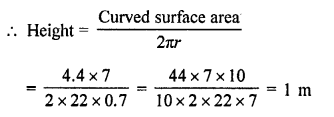Question 2 : In a hot water heating system, there is a cylindrical pipe of length 28 m and diameter 5 cm. Find the total radiating surface in the system. [NCERT]

Answer 2 : Diameter of the pipe = 5 cm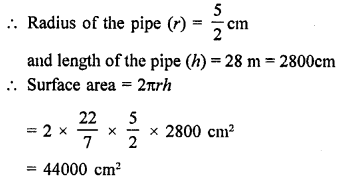Question 3 : A cylindrical pillar is 50 cm in diameter and 3.5 m in height. Find the cost of painting the curved surface of the pillar at the rate of 12.50 per m2. [NCERT]

Answer 3 : Diameter of cylindrical pillar = 50 cm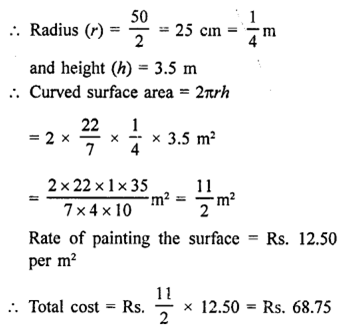Question 4 : It is required to make a closed cylindrical tank of height 1 m and base diameter 140 cm from a metal sheet. How many square metres of the sheet are required for the same? [NCERT]

Answer 4 : Height of cylinder (h) = 1 m = 100 cm
Diameter of box = 140 cm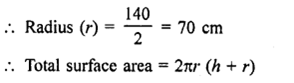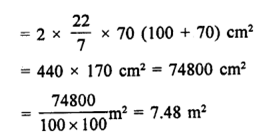Question 5 : The total surface area of a hollow cylinder which is open from both sides is 4620 sq. cm, area of base ring is 115.5 sq. cm and height 7 cm. Find the thickness of the cylinder.

Answer 5 : Total surface area of a hollow cylinder open from bothsides = 4620 cm2
Area of base of ring = 115.5 cm2
Height (h) = 7 cm
Let outer radius (R) = R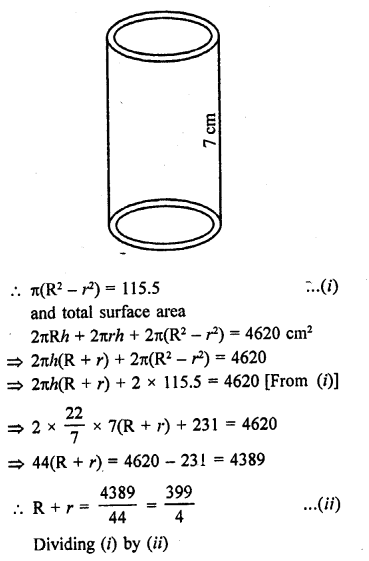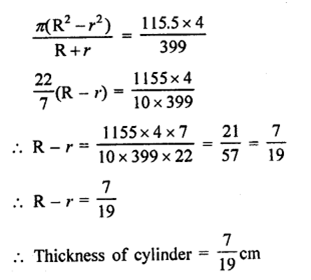Question 6 : Find the ratio between the total surface area of a cylinder to its curved surface area, given that its height and radius are 7.5 cm and 3.5 cm.

Radius of the cylinder (r) = 3.5 cm
and height (h) = 7.5 cm
Total surface area = 2πr (h + r)
and curved surface area = 2πrh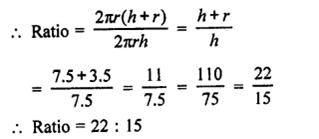Question 7 : A cylindrical vessel, without lid, has to be tin-coated on its both sides. If the radius of the base is 70 cm and its height is 1.4 m, calculate the cost of tin-coating at the rate of ₹3.50 per 1000 cm2.

Answer 7 : Radius of the base of a cylindrical vessel (r) = 70 cm
and height (h) = 1.4 m = 140 cm
Total surface area (excluding upper lid) on both sides = 2πrh x 2 + πr2 x2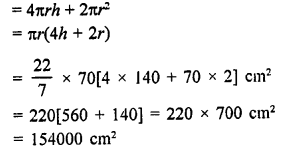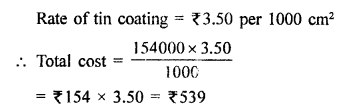Question 8 :
The inner diameter of a circular well is 3.5 m. It is 10 m deep. Find:
(i) inner curved surface area.
(ii) the cost of plastering this curved surface at the rate of ₹40 per m2. [NCERT]

Answer 8 : Inner diameter of a well = 3.5 m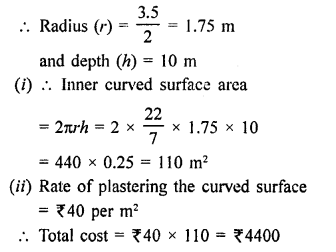Question 9 : The students of a Vidyalaya were asked to participate s a competition for making and decorating pen holders in the shape of a cylinder with a base, using cardboard. Each pen holder was to be of radius 3 cm and height 10.5 cm. The Vidyalaya was to supply the competitors with cardboard. If there were 35 competitors, how much cardboard was required to be bought for the competition? [NCERT]

Answer 9 : Radius of cylinderical pen holder (r) = 3 cm
Height (h) = 10.5 cm
Surface area of the penholder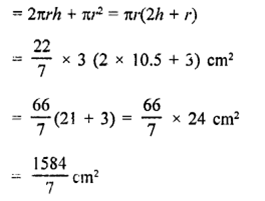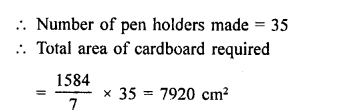Question 10 : The diameter of roller 1.5 m long is 84 cm. If it takes 100 revolutions to level a play¬ground, find the cost of levelling this ground at the rate of 50 paise per square metre.

Answer 10 : Diameter of a roller = 1.5 m
Radius = 1.5/2 = 0.75 m = 75 cm
and length (h) = 84 cm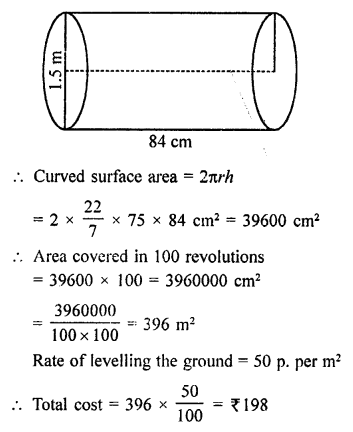Todays Deals### RD Chapter 19- Surface Areas and Volume of a Circular Cylinder Ex-19.1 Contributorskrishan

Name:
Email:

# Latest News# 9000 interview questions in different categories